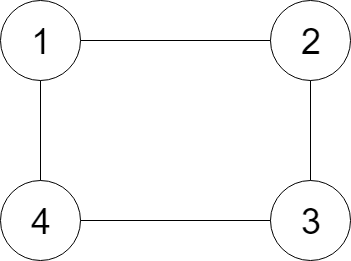# LeetCode – Clone Graph

### 题目：

Given a reference of a node in a connected undirected graph, return a deep copy (clone) of the graph. Each node in the graph contains a val (`int`) and a list (`List[Node]`) of its neighbors.

Example:Input:

```{"\$id":"1","neighbors":[{"\$id":"2","neighbors":[{"\$ref":"1"},{"\$id":"3","neighbors":[{"\$ref":"2"},{"\$id":"4","neighbors":[{"\$ref":"3"},{"\$ref":"1"}],"val":4}],"val":3}],"val":2},{"\$ref":"4"}],"val":1}

```

Explanation:

```Node 1's value is 1, and it has two neighbors: Node 2 and 4.
Node 2's value is 2, and it has two neighbors: Node 1 and 3.
Node 3's value is 3, and it has two neighbors: Node 2 and 4.
Node 4's value is 4, and it has two neighbors: Node 1 and 3.
```

Note:

1. The number of nodes will be between 1 and 100.
2. The undirected graph is a simple graph, which means no repeated edges and no self-loops in the graph.
3. Since the graph is undirected, if node p has node q as neighbor, then node q must have node p as neighbor too.
4. You must return the copy of the given node as a reference to the cloned graph.

### 解题：

• 深度优先DFS：

使用递归方式，利用hashmap建立原节点和克隆节点的对应关系， 判断是否已经进行克隆， 处理过程：

1. 先判断结束条件： 当前节点是否为空；
2. 判断为非空节点后， 通过hashmap判断是否克隆过， 发现已经克隆过，直接反馈其克隆节点；
3. 对于未进行克隆的节点，对当前节点进行克隆， 并且在hashmap中建立映射关系；
4. 遍历当前节点所有neighbors 中的节点，再次调用cloneGraph函数，将他们克隆到当前节点克隆节点的neighbors数组中。
5. 返回克隆节点；

### 实现：

``````// Definition for a Node.
class Node {
public:
int val;
vector<Node*> neighbors;

Node() {}

Node(int _val, vector<Node*> _neighbors) {
val = _val;
neighbors = _neighbors;
}
};
*/
/* DFS */
class Solution {
public:
Node* cloneGraph(Node* node) {
if(!node){
return NULL;
}
if (copies.find(node) == copies.end()){
copies[node] = new Node(node->val, {});
for (Node* neighbor : node->neighbors){
copies[node]->neighbors.push_back(cloneGraph(neighbor));
}
}
return copies[node];
}
private:
unordered_map<Node*, Node*> copies;
};``````
• 广度优先BFS：

增加队列queue进行辅助，利用hashmap建立原节点和克隆节点的对应关系，判断是否已经进行克隆，处理过程：

1. 先判断结束条件： 当前节点是否为空；
2. 判断为非空节点后，对当前节点进行克隆， 并且在hashmap中建立映射关系，并将其放入队列queue；
3.  对队列进行处理，取出队列的元素。
4.  遍历其所有的neighbors数组的节点neighbor， 如果不在hashmap中，克隆一个新的neighbor节点，并在hashmap中建立映射关系， 将其放入队列，并将其克隆到当前节点克隆节点的neighbors数组中
5. 重复3，4处理，直到队列为空退出。
6. 返回克隆节点；
``````// Definition for a Node.
class Node {
public:
int val;
vector<Node*> neighbors;

Node() {}

Node(int _val, vector<Node*> _neighbors) {
val = _val;
neighbors = _neighbors;
}
};
//BFS
class Solution {
public:
Node* cloneGraph(Node* node) {
if(!node){
return NULL;
}
Node *copy = new Node(node->val, {});
copies[node] = copy;
queue<Node*> q;
q.push(node);
while (!q.empty()){
Node* cur = q.front();
q.pop();
for (Node* neighbor : cur->neighbors){
if (copies.find(neighbor) == copies.end()){
copies[neighbor] = new Node(neighbor->val, {});
q.push(neighbor);
}
copies[cur]->neighbors.push_back(copies[neighbor]);
}
}

return copies[node];
}
private:
unordered_map<Node*, Node*> copies;
};``````